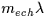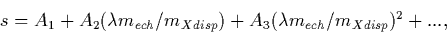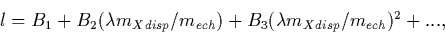Next: 8.3.2 Calculation of the Up: 8.3 High-Dispersion Wavelength Calibration Previous: 8.3 High-Dispersion Wavelength Calibration

8.3.1 Parameterization of Dispersion Relations

The separation of the echelle orders in the spatial direction by the cross-disperser complicates the calculation of the wavelength parameterization of high-dispersion IUE spectra. NEWSIPS departs from IUESIPS in seeking a 1:1 correspondence between dispersion parameters and physical properties of the spectro-optical system. The goal of such a representation is to identify each term with optical properties of the spectrograph and to prevent these physical effects from introducing cross-terms that could complicate the estimate of errors in the wavelength solution (for a full discussion of this concept, see Smith 1990a, 1990b). In the raw geometry this is not possible because the rotation of the order produces a correlation of the line and sample positions for a wavelength. For low-dispersion images this problem is largely solved by de-rotating the image. In the high-dispersion geometry the dispersion axis is also dependent on the echelle order: the precise angle is equal to the tangent of the dispersing powers of the echelle and cross-dispersing grating. Because this factor varies as 1/mech, the dispersion axis slowly rotates and produces a splaying of the orders (see Chapter 7.3.1). In order to place all echelle orders along a common pseudo-dispersion axis, the order-splaying is removed as part of a single resampling step in the GEOM module. This removes the second and last of the important cross-coupling terms between the two axes in the original raw space. Thus the GEOM resampling forces the echelle orders to fall along a common sample axis (s) and to be separated by a difference in line positions (l) on the high-dispersion SI. This operation produces a small tilt of a monochromatic image on an order which is usually ignored because its effect on the spectral instrumental profile is small.

The representation of the dispersion parameters in the rectilinear (s, l) high-dispersion SI geometry can be expressed as a Taylor expansion of the grating equation in terms of the quantity.The equations for the dispersion solution for sample and line positions are:(2)(3)
where the m's refer to the order numbers for the echelle and cross-dispersing gratings (mXdisp = 1) and the A's and the B's are constants to be determined empirically. The relations in Equations 8.1 and 8.2 represent an orthogonal system (i.e., each of the factors is decoupled from the others).

In practice higher-order terms in the Taylor expansion of the dispersion solution are small for the IUE gratings and need not be considered. However, the quadratic term is still significant for the IUE grating geometry (Smith 1990a, 1990b). Furthermore, although the cubic and quartic terms are not significant in the Taylor expansion of the grating equation, such terms do arise from the electro-optical distortions within the IUE cameras. These high-order terms (quadratic, cubic, and quartic) have been determined empirically as a function of echelle order and incorporated within the GEOM module as additional terms, as in the linearization process performed in low dispersion (Chapter  8.1). The result of these GEOM corrections is an SI with very nearly linear relation between wavelength and sample position in each order.Next: 8.3.2 Calculation of the Up: 8.3 High-Dispersion Wavelength Calibration Previous: 8.3 High-Dispersion Wavelength Calibration
Karen Levay
12/4/1997